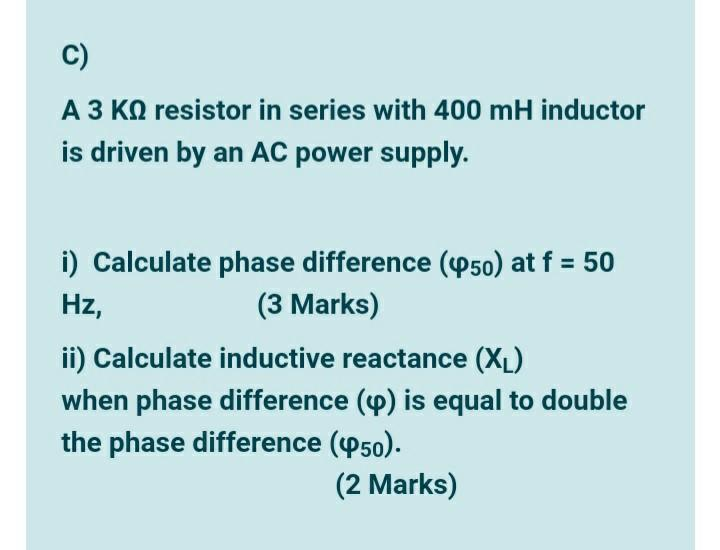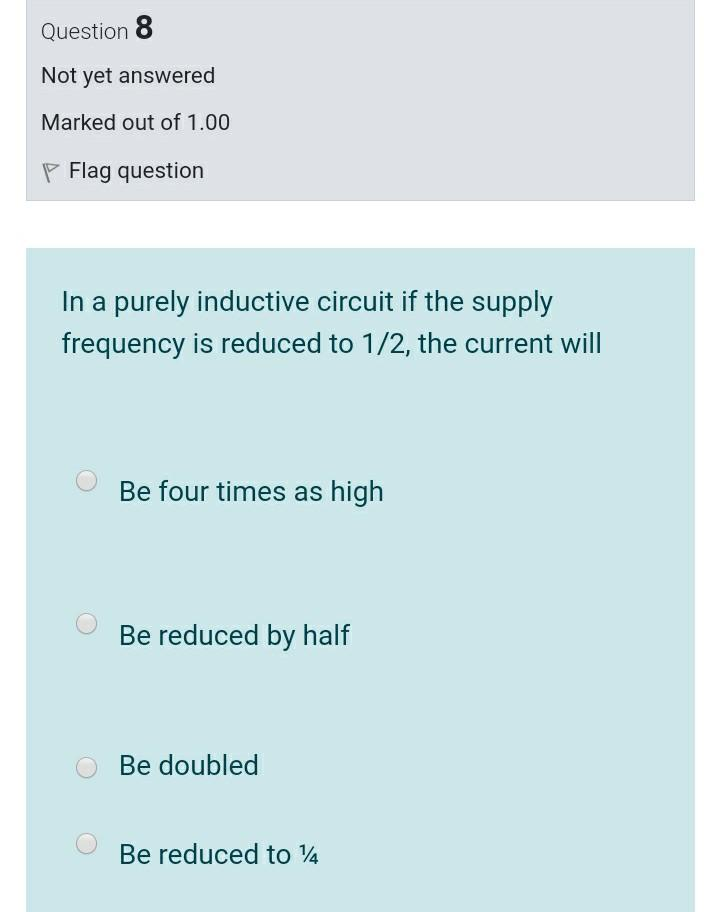### Create an Account

Home / Questions / A 3 K9 resistor in series with 400 mH inductor is driven by an AC power supply. Hz, i) Cal...

# A 3 K9 resistor in series with 400 mH inductor is driven by an AC power supply. Hz, i) Calculate phase difference (450) at f = 50 ii) Calculate inductive reactance (XL) when phase difference

3 K9 resistor in series with 400 mH inductor is driven by an AC power supply. Hz, i) Calculate phase difference (450) at f = 50 (3 Marks) ii) Calculate inductive reactance (XL) when phase difference (9) is equal to double the phase difference (950). (2 Marks)
Question 8 Not yet answered Marked out of 1.00 P Flag question In a purely inductive circuit if the supply frequency is reduced to 1/2, the current will Be four times as high Be reduced by half Be doubled Be reduced to 14May 31 2021 View more View LessSubscribe To Get Solution